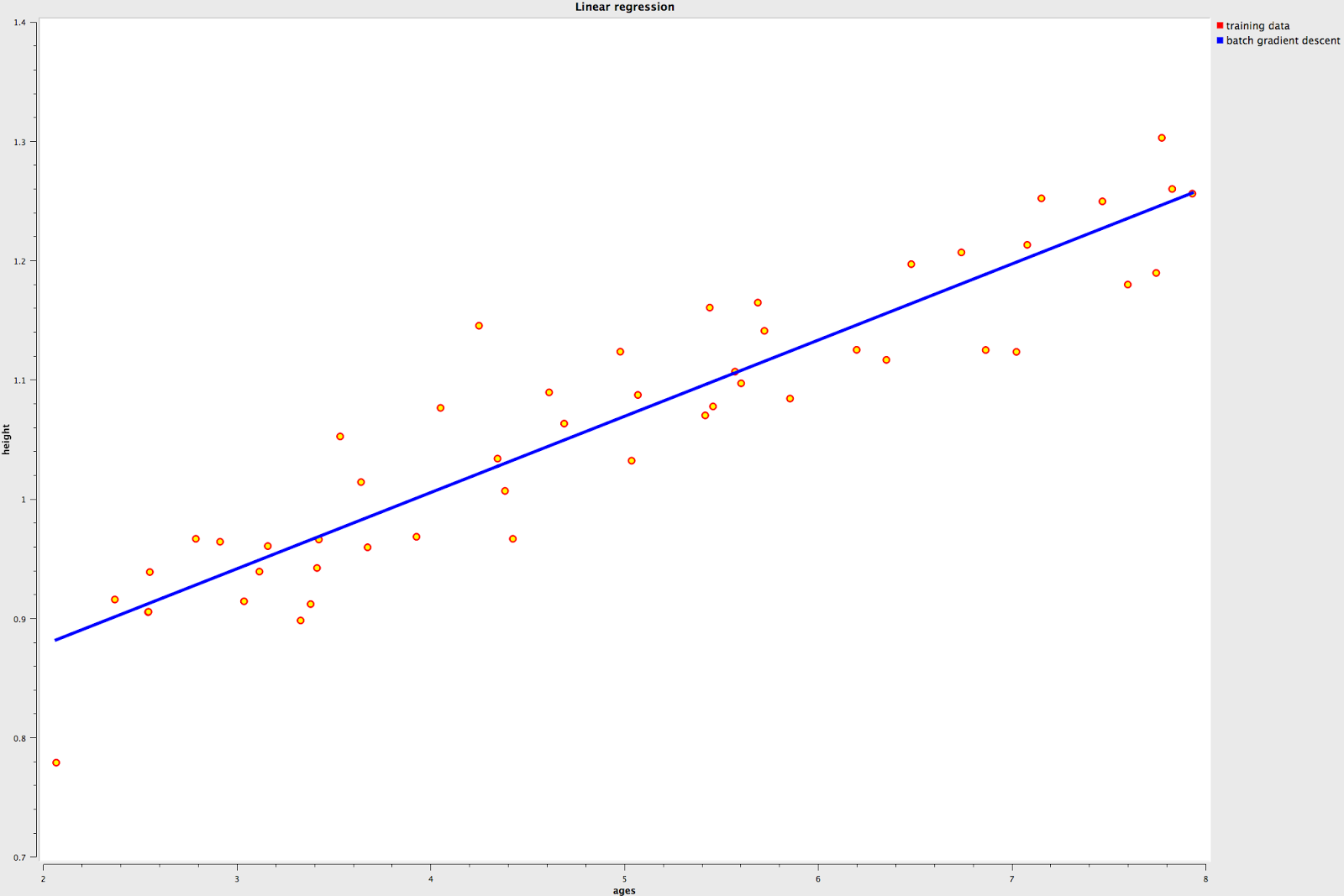## Thursday, 7 November 2013

### machine learning--00 : supervised vs unsupervised

Machine learning is a very important and practical skills in computer vision, in order to make a good use of the machine learning algorithm provided by openCV,  I decided to study machine learning and record the things I learned from the open classroom of standford.

There are two major branch of machine learning algorithms, they are supervised and unsupervised learning.

Supervised learning : teach the program what is the correct answers, the input will contain "features" and "labels".

Unsupervised learning : let the program learn by itself, the input contain "features" but no "labels".

Example of Supervised learning

Height prediction : There are various numbers of boys between ages(features) of two and eights, we want to predict the heights(labels) of different ages.graph-00 : ages(x-axis) vs height(y-axis) without predict

On graph-00,  I plot the ages vs height with dot,  the range of ages are analog but not digital(2.54 years old, 3.33 years old and so on), how could we predict the height of age 4.44 which do not shown on this graph? This is the time when machine learning comes in,  we give the program features(in this case, it is ages) and labels(in this case, it is height), use them to deduce an equation which could map arbitrary ages within 2~8 years old, we call this equation as hypothesis function and make it at-least close to the result of the data set we have.graph-01 : ages(x-axis) vs height(y-axis) with predict(blue line)
On graph-01, I map the ages to height by the equation deduce by the batch gradient descent, since the ranges of the labels are analog but not digital, this kind of supervised learning also refer to regression.

The other examples is image recognition, like the simple OCR engine developed by this blog also leverage the power of supervised learning to predict the character of the image.Because the results are discrete in the OCR example, we also refer that kind of supervised learning as classification.

Example of unsupervised learning

k-means is one of the unsupervised machine learning algorithm, you give it a bunch of data without telling the program which label the data belong to, let the program determine which label of those data belong to, this also called clustering.graph_02 : before k-means clusteringgraph_03 : after k-means clustering